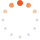# Tistory

티스토리 메뉴 펼치기

티스토리 메뉴 펼치기 댓글수1

프로그래밍/기타

## [프로그래머스][Python3] 단어 변환

snoopybox
댓글수1

문제 :

https://programmers.co.kr/learn/courses/30/lessons/43163

나의 풀이 :

from collections import deque

def solution(begintargetwords):
def is_only_one_diff(ab):
remain = 1
for i in range(len(a)):
if a[i] != b[i]:
remain -= 1
if remain < 0:
return False
return remain == 0

queue = deque([(begin, 0)])
visited = set()
while queue:
curr, depth = queue.popleft()
if curr == target:
return depth
for word in words:
if is_only_one_diff(curr, word) and word not in visited:
queue.append((word, depth+1))
return 0

나의 풀이2 :

deque를 사용하지 않는 버전

def solution(begintargetwords):
def is_only_one_diff(ab):
remain = 1
for i in range(len(a)):
if a[i] != b[i]:
remain -= 1
if remain < 0:
return False
return remain == 0

queue = [begin]
depth = 0
visited = set()
while queue:
next_queue = set()
for curr in queue:
if curr == target:
return depth